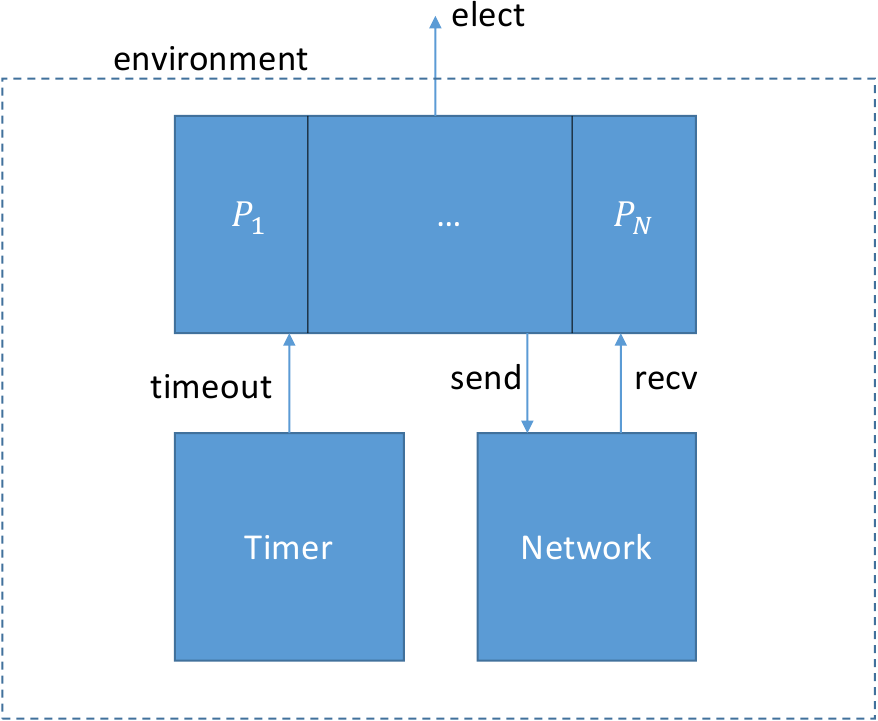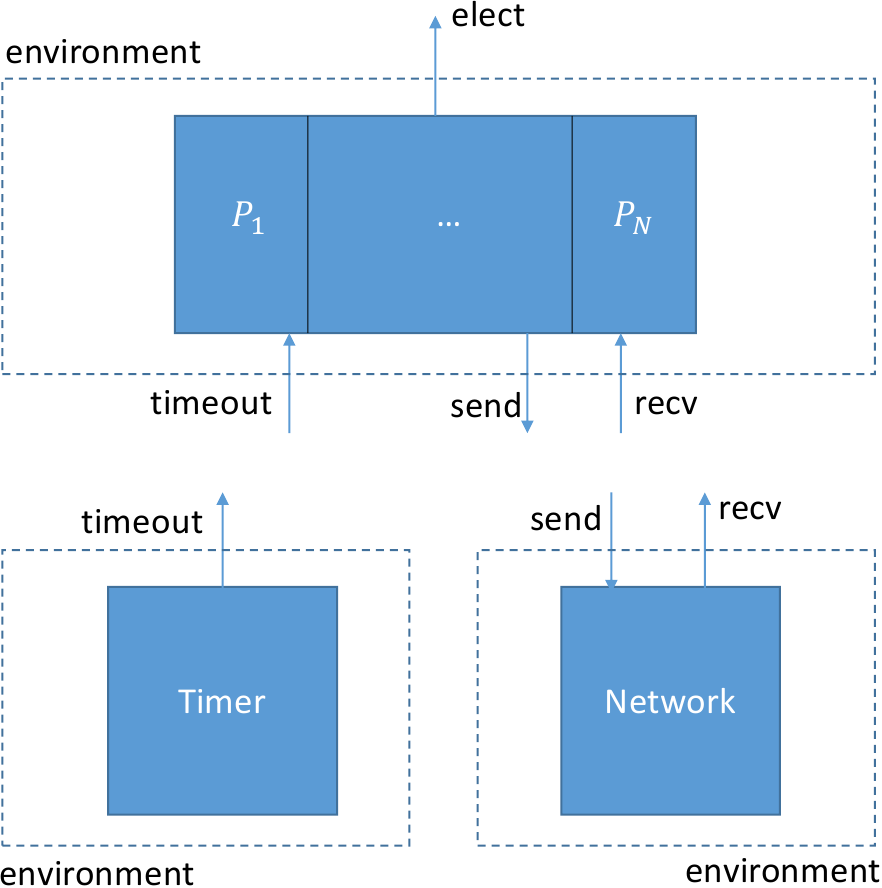We just saw a very simple example of compositional testing of a peer-to-peer interface. It’s also possible to use compositional testing at the interface between layers in a layered protocol stack.

As an example, let’s look at the very simple leader election protocol, introduced in this paper in 1979.

In this protocol we have a collection of distributed processes organized in a ring. Each process can send messages to its right neighbor in the ring and receive message from left neighbor. A process has a unique `id` drawn from some totally ordered set (say, the integers). The purpose of the protocol is to discover which process has the highest `id` value. This process is elected as the “leader”.

This protocol itself is trivially simple. Each process transmits its own `id` value. When it receives a value, it retransmits the value, but only if it is greater than the process’ own `id` value. If a process receives its own `id`, this value must have traveled all the way around the ring, so the process knows its `id` is greater than all others and it declares itself leader.

# Interface specifications

``````object asgn = {

function pid(X:node.t) : id.t
axiom [injectivity] pid(X) = pid(Y) -> X = Y
}

object serv = {

action elect(v:node.t)

object spec = {
before elect {
assert asgn.pid(v) >= asgn.pid(X)
}
}
}
``````

The abstract type `node.t` represents a reference to a process in our system (for example, its network address). The `asgn` object defines an assignment of `id` values to nodes, represented by the function `pid`. The fact that the `id` values are unique is guaranteed by the axiom `injectivity`.

The service specification `serv` defines one action `elect` which is called when a given node is elected leader. Its specification says that only the node with the maximum `id` value can be elected.

Our protocol consists of a collection of concurrent process layered on top of two services: a network service and a timer service:Let’s consider now the two intermediate interfaces. The specification for the network service is quite simple and comes from IVy’s standard library:

``````module ip_simple(addr,pkt) = {

object spec = {
after init {
sent(V, N) := false
}

before send {
sent(v,dst) := true
}
before recv {
assert sent(v,dst)
}
}
}
``````

The interface is a module with two parameters: the type `addr` of network addresses, and the type `pkt` of packets. It has two actions, `recv` and `send`. The relation `sent` tells us which values have been sent to which nodes. Initially, nothing has been sent. The interface provides two actions `send` and `recv` which are called, respectively, when a value is sent or received. The `src` and `dst` parameters respectively give the source and desitination addresses of the packets.

The specification says that a value is marked as sent when `send` occurs and a value must be marked sent before it can be received. This describes a network service that can duplicate or re-order messages, but cannot corrupt messages.

The specification of the timer service is also quite simple and comes from the standard libarary:

``````module timeout_sec = {

import action timeout

}
``````

The timer service simply calls `timeout` from time to time, with no specification as to when.

# Protocol implementation

Now that we have the specificaitons of all the interfaces, let’s define the protocol itself:

``````instance net : ip_simple(node.t,id.t)
instance timer(X:node.t) : timeout_sec

object proto = {

implement timer.timeout(me:node.t) = {
call trans.send(node.next(me),asgn.pid(me))
}

implement net.recv(me:node.t,v:id.t) {
if v = asgn.pid(me) {       # Found a leader!
call serv.elect(me)
}
else if v > asgn.pid(me)  { # pass message to next node
call trans.send(node.next(me),v)
}
}

}
``````

We create one instance of the network module, and one timer for each node. The protocol implements the interface actions `timer.timeout` and `net.recv`. When a node times out, it transmits the node’s `id` to the next process in the ring, defined by the the action `node.next` (which we’ll define shortly).

When a node receives an id value `v`, it checks whether `v` is its own id according to the id assignment `asgn`. If so, the process knows it is leader and calls `serv.elect`. Otherwise, if the received value is greater, it calls `net.send` to send the value on to the next node in the ring.

With our protocol implemented, we still need to provide concrete types for `node` and `id`. If we were formally verifying the protocol, we would probably treat these as abstract datatypes with specified mathematical properties. For testing, though, we just need some concrete types. Here is one possiblity:

``````object node = {
type t
interpret t -> bv

action next(x:t) returns (y:t) = {
y := x + 1
}
}

object id = {
type t
interpret t -> bv
}
``````

That is, node addresses are one-bit binary numbers (meaning we have just two nodes, and id’s are 8-bit numbers. Often it’s a good idea to start testing with small datatypes. In the case of the leader election ring, more nodes would increase the amount of time needed to see an election event.

## Compositionally testing the leader election protocol

We’re going to divide the system into three isolates, one for each of its major components. Here is what the three isolates will look like:Here are the isolate definitions:

``````trusted isolate iso_p = proto with serv,node,id,asgn,net,timer
trusted isolate iso_t = timer
trusted isolate iso_n = net with node,id
``````

We added the keyword `trusted` to these isolates to indicate to IVy that they will be verified informally by testing. Notice that the isolate for `proto` contains its service specification as well as the specifications of all of the other components. We need to test that `proto` lives up to its guarantees at all of its interfaces, provided that all of its assumptions hold. On the other hand, `net` needs only the specifications of `node` and `id` (the `net` object contains its own service specification).

We can now use IVy to compile test environments for each of the isolates, based on the interface specifications. Let’s start with the high-level protocol:

``````\$ ivy_to_cpp isolate=iso_p target=test build=true leader_election_ring.ivy
``````

We run the tester:

``````\$ ./leader_election_ring
> timer.timeout(1)
< net.send(1,0,170)
> net.recv(0,170)
< net.send(0,1,170)
> net.recv(0,170)
< net.send(0,1,170)
> net.recv(0,170)
< net.send(0,1,170)
> timer.timeout(0)
< net.send(0,1,149)
> timer.timeout(0)
< net.send(0,1,149)
> timer.timeout(0)
< net.send(0,1,149)
> net.recv(0,170)

...

> net.recv(0,170)
< net.send(0,1,170)
> timer.timeout(0)
< net.send(0,1,149)
> net.recv(1,170)
< serv.elect(1)
``````

In this run, process 1 times out and sends its id 170 to process

1. Process 0 receives this id and forwards it back to process 1 (meaning process 0’s id must be less than 170). Later, process 0 times out and sends its id 149 to process 1. Eventually, the network decides to deliver to process 1 the message with its own id, causing process 1 to be elected. When this happens, the assertion monitor checkes that in fact process 1 has the highest id.

The important point here is that there is no actual network. The tester is simulating the network by randomly selecting actions consistent with the history of actions at the interface. In practice, this means that the tester stores the relation `sent` (the state of the network interface specification) and only calls `net.recv` with packets that have been previously sent. When there are multiple choices, the tester makes an effort to select uniformly. This is a hard problem in general, however. In practice the distribution will be non-uniform. If you look at the calls to `net.recv`, you can see that there is some duplication of sent packets, as we would expect.

We can see here a major advantage of compositional testing over integration testing. If we used a real network to test our protocol, we might have to wait a long time for a packet duplication to occur (in fact, this might never occur unless we take care to stress the network appropriately). The resilience of our protocol to packet duplication would not be well tested, as it is here. Similarly, our protocol might have unintended dependences on timing. Since timeout events are being generated randomly here, and not based on wall clock time, we can see timings that might occur rarely in the actual system, perhaps only under very heavy load.

Now let’s turn to the network isolate. We compile and run:

``````\$ ivy_to_cpp isolate=iso_n target=test build=true leader_election_ring.ivy

> net.send(0,0,168)
< net.recv(0,168)
> net.send(0,0,243)
< net.recv(0,243)
> net.send(0,0,13)
< net.recv(0,13)
> net.send(1,0,108)
> net.send(0,0,84)
> net.send(0,0,78)
< net.recv(0,108)
< net.recv(0,84)
< net.recv(0,78)
> net.send(1,0,17)
< net.recv(0,17)
> net.send(0,1,167)
< net.recv(1,167)
> net.send(1,0,3)
> net.send(0,1,13)
...
``````

The object `net` is actually just a wrapper around the operating system’s networking API. Therefore, when we test `net`, we are actually testing the operating system’s networking stack. Fortunately, testing reveals no errors. Notice though, that the tester is generating a wider variety of packets than the protocol could actually generate. In fact, it’s simply generating packet values uniformly at random. In this sense, the network stack is also being better tested than it would be if composed with the actual protocol.

Finally, we can test the timer:

``````\$ ivy_to_cpp isolate=iso_t target=test build=true leader_election_ring.ivy

< timer.timeout(0)
< timer.timeout(1)
< timer.timeout(0)
< timer.timeout(1)
< timer.timeout(0)
< timer.timeout(1)
< timer.timeout(0)
< timer.timeout(1)
< timer.timeout(0)
...
``````

Not very interesting, but it shows both timeouts occurring once every second, which is what we expect. Again, we are really testing the operating system here (and also IVy’s run time scheduler, which uses the operating system API).

# Running the protocol

Now that we’ve tested the components, we can compile the whole system and run it as a collection of independent processes. Our protocol is an example of a parameterized object. Ivy can decompose it into a collection of processes based on the parameter `me`. Each value of the parameter becomes an individual process. This transformation is called parameter stripping.

To do this, we use the following declaration:

``````extract iso_impl(me:node.t) = proto(me),net(me),timer(me),node,id,asgn
``````

This describes a special kind of isolate called an `extract`. Extracts are intended to be run in production and don’t include the specification monitors. In the extract declaration, `me` is a symbolic constant that represents the first parameter of of each action and state component in the object. This parameter is “stripped” by replacing it with the symbolic constant `me`.

Before thinking about this too hard, let’s just run it to see what happens. We compile as usual, with one difference: we use the option `target=repl`. Instead of generating a test environment, IVy provides a read-eval-print loop as the environment:

``````\$ ivy_to_cpp target=repl isolate=iso_impl build=true leader_election_ring.ivy
``````

This produces an executable that takes one parameter `me` on the command line. Let’s create two terminals A and B to run process 0 and process 1 respectively:

``````A: \$ ./leader_election_ring 0
A: >